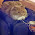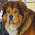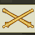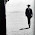## Saturday, January 05, 2013

### Ok braniacks

The FB comments were pretty much equal on two different numbers.

1.20.

Order of operations, bitchez.

1.There was another just about as popular.

2.I'm with bluesun. It's an order of operations question and the correct answer is 20.

Order of operations is exponents/roots, then multiplication/division, then Addition/subtraction always work inside parentheses before working outside them.
Let's restate the problem using parentheses to point to the first operations
4x4+4x4+4-4x4=

can be restated to be

(4x4)+(4x4)+(4)-(4x4)=

which is

16+16+4-16=

which is the same as

16+4 = 20

3.20 here too. (16)+(16)-(16)+(4). What the heck would anyone else say?

4.20.....I'm trying to figure out what other answer would be the big one lol

5.Although I get 20, I'll bet most people don't remember order of operations and get 320.

1.Yep- 320 was the other one.

I got twenty in about three seconds.

6.Please excuse my dear aunt Sally.

7.8.20 is correct.

9.Yep, higher math is done first.

10.11.20.

Order of operations: My Dear Aunt Sally (multiply, divide, add, subtract)

Or plug it into an Excel cell if you don't believe me.

MC

12.13.I got 320.

I must have been drunk the day they taught order of operations.

14.15.16.